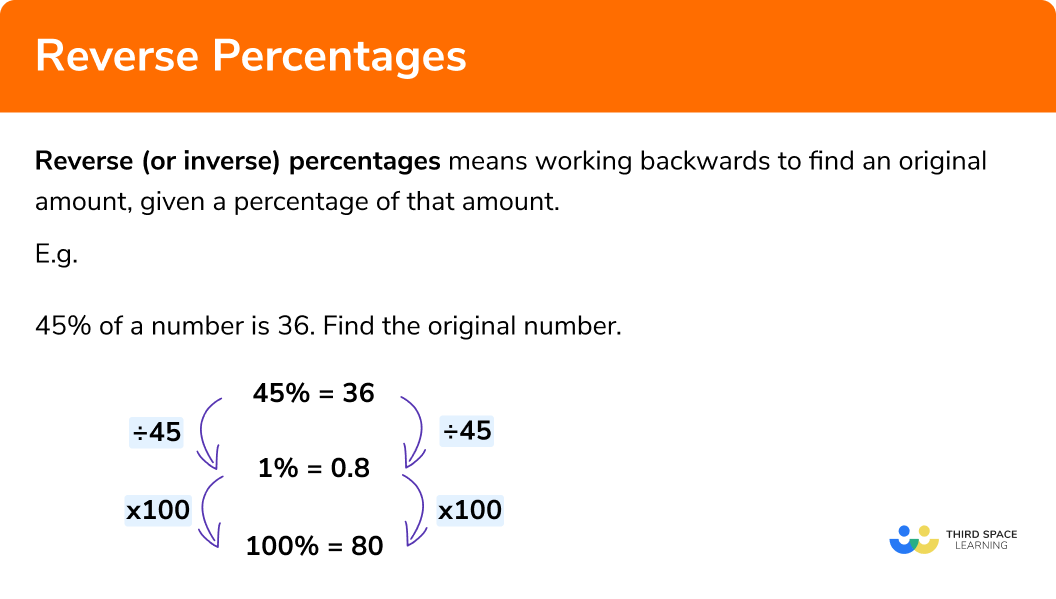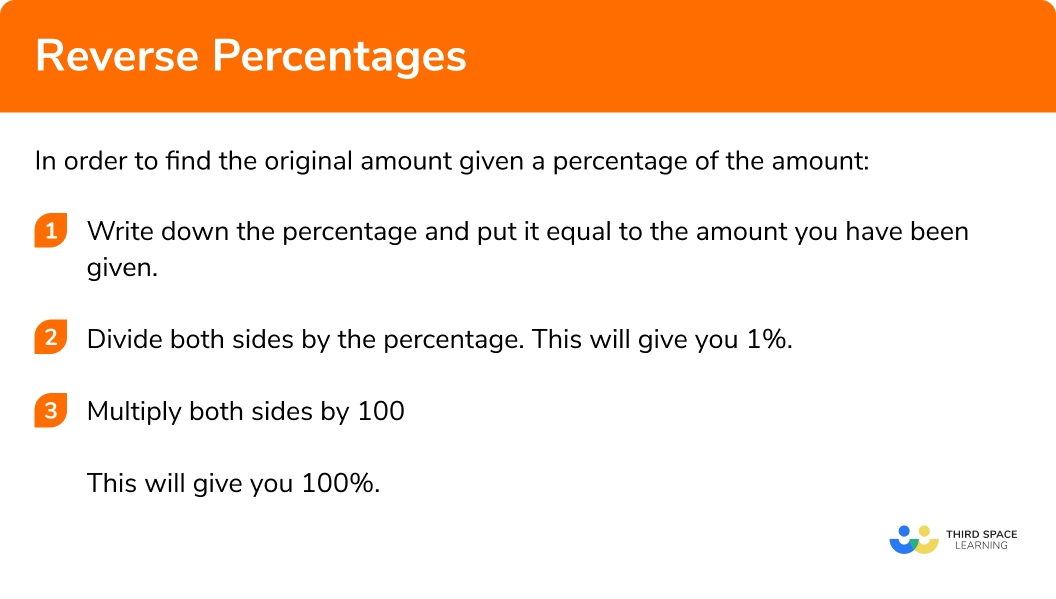GCSE Maths Number FDP Percentages

Reverse Percentages

# Reverse Percentages

Here we will learn about reverse percentages including how to work backwards to find an original amount given a percentage of that amount or a percentage increase/decrease.

There are also reverse percentages worksheets based on Edexcel, AQA and OCR exam questions, along with further guidance on where to go next if you’re still stuck.

## What are reverse percentages?

Reverse percentages (or inverse percentages) means working backwards to find an original amount, given a percentage of that amount.

• We can do this using a calculator by taking the percentage we have been given, dividing to find 1% and then multiplying by 100 to find 100%.
• We can also do this without a calculator by using factors of the percentage we have been given.
• Sometimes we are given a percentage of an amount and we need to work out what the original value was.

We need to remember that the original amount is 100% of the value.

### What are reverse percentages?## How to use reverse percentages given a percentage of an amount (calculator method)

In order to find the original amount given a percentage of the amount (using a calculator):

1. Write down the percentage and put it equal to the amount you have been given.
2. Divide both sides by the percentage.
(e.g. if you have 80%, divide both by 80). This will give you 1%.

3. Multiply both sides by 100.
This will give you 100%.

### Explain how to find the original amount given a percentage of the amount in 3 steps## Reverse percentageexamples (calculator method)

### Example 1: calculator

45% of a number is 36. Find the original number.

1. Put the percentage equal to the amount.

45% = 36

2 Divide both sides by the percentage to find 1%.

In this case the percentage is 45%, so divide by 45.

3 Multiply by 100 to find 100%.

The original number was 80.

### Example 2: calculator

150% of a number is 690. Calculate the original number.

150% = 690

The original number was 460.

## How to use reverse percentages given a percentage of an amount (non-calculator method)

In a situation where we do not have a calculator, we can often simplify the problem by using common factors.
Rather than finding 1%, which might involve a difficult division, we could find 10%, 25%, or any other percentage which is a factor of 100%.

1. Write down the percentage and put it equal to the amount you have been given.
2. Identify a common factor of the percentage and 100% (a number which goes in to both).
3. Use division to find that percentage of your amount.
4.  Use multiplication to find 100%.

## Reverse percentages examples (non-calculator method)

### Example 3: non-calculator

70% of an amount is 56. Find the original amount.

70% = 56

Factors of 70: 1, 2, 5, 7, 10, 14, 35, 70

Factors of 100: 1, 2, 4, 5, 10, 20, 25, 50, 100

Here 1, 2, 5 and 10 are all factors of both 70 and 100. We could use any of these but select the one which results in the easiest calculations (usually the highest common factor). In this case we are going to use 10%.

The original amount was 80.

Note: In this example, and every example, the method of finding % would also work. The reason that we found 10% here rather than 1% is that 56 ÷ 7 is easier to work out without a calculator than 56 ÷ 70.

### Example 4: non-calculator

125% of a number is 350. Find the original value.

125% = 350

Factors of 125: 1, 5, 25, 125

Factors of 100: 1, 2, 4, 5, 10, 20, 25, 50, 100

Here we are going to use 25%.

The original amount was 280.

## How to use reverse percentages given a percentage increase/decrease

Sometimes, instead of being told a percentage of the amount, we are told what percentage increase or decrease has occurred.
The only difference here compared to what we have already looked at is that we first need to identify what percentage of the original amount we now have.

1. Identify what percentage of the original amount you now have.
If it has been increased by a percentage, add that percentage onto 100%.

If it has been decreased by a percentage, subtract that percentage from 100%.
2. Write down the percentage and put it equal to the amount you have been given.
3. Use either the calculator or non-calculator method to find 100%.

## Reverse percentages examples (percentage increase/decrease)

### Example 5: percentage increase, calculator

The number of fans attending a football match this week was 12% more than last week. If 728 people attended the match this week, how many attended last week?

100% + 12% = 112%

112% = 728

The number of fans last week was 650.

### Example 6: percentage decrease, calculator

The value of a car has decreased by 16.5% in the last year. If its value now is £5845, find its original price.

100%16.5% = 83.5%

83.5% = 5845

The original price was £7000.

### Example 7: percentage increase, non-calculator

A puppy’s weight has increased by 20% to 4.8kg. What was the puppy’s weight before the increase?

100% + 20% = 120%

120% = 4.8

Factors of 120: 1, 2, 3, 4, 5, 6, 8, 10, 12, 15, 20, 24, 30, 40, 60, 120

Factors of 100: 1, 2, 4, 5, 10, 20, 25, 50, 100

There are several common factors here. It would be easiest to use 10% or 20%. Here we are going to use 20%.

The puppy’s original weight was 4kg.

### Example 8: percentage decrease, non-calculator

A television is in a 10% sale. The sale price of the television is £450. Find the original price of the television.

100%10% = 90%

90% = 450

Factors of 90: 1, 2, 3, 5, 6, 9, 10, 15, 30, 45, 90

Factors of 100: 1, 2, 4, 5, 10, 20, 25, 50, 100

Here we are going to use 10%.

The original price of the television was £500.

### Common misconceptions

• Calculating a percentage and adding it on

A common mistake is to work out the percentage of the number and then add it on.
E.g.
Given 70% of a number, a common error is to calculate 30% of that number to add on to the 70%.
Remember, this does not work as 30% of 70% would not be the same as 30% of the original value.

• Not adding/subtracting from 100% when it is a percentage increase/decrease

A common mistake is to use the actual percentage increase/decrease rather than adding/subtracting from 100%.
E.g
If you are told it is a 30% decrease, a common error would be to use 30% instead of 70%.

Reverse percentages is part of our series of lessons to support revision on percentages. You may find it helpful to start with the main percentages lesson for a summary of what to expect, or use the step by step guides below for further detail on individual topics. Other lessons in this series include:

### Practice reverse percentages questions

You may use a calculator for questions 1, 2 and 5.

1. 36\% of a number is 324 . Find the original number.

117900360116636\% = 324

Dividing both sides by 36 gives

1\% = 9

Multiplying both sides by 100 gives

100\% = 900

2. 145\% of a number is 2900. Find the original number.

3045275520001885145\% = 2900

Dividing both sides by 145 gives

1\% = 20

Multiplying both sides by 100 gives

100\% = 2000

3. 60\% of a number is 210. Find the original number.

12652527035060\% = 210

Dividing both sides by 3 gives

20\% = 70

Multiplying both sides by 5 gives

100\% = 350

4. 150\% of a number is 33. Find the original number.

442249.511150\% = 33

Dividing both sides by 3 gives

50\% = 11

Multiplying both sides by 2 gives

100\% = 22

5. The price of a car is reduced by 15\%. The reduced price is £6800. Find the original price.

£8000£7820£5780£6785The price has been reduced by 15\%, so £6800 is equal to 85\% of the original price.

85\% = £6800

Dividing both sides by 85 gives

1\% = 80

Multiplying both sides by 100 gives

100\% = 8000

6. The number of customers who visited a shop today was 10\% higher than the number who visited yesterday. Today 231 customers visited the shop. How many customers visited the shop yesterday?

221210241218The number of customers has increased by 10\%, so 231 is equal to 110\% of the number from yesterday.

110\% = 231

Dividing both sides by 11 gives

10\% = 21

Multiplying both sides by 10 gives

100\% = 210

### Reverse percentagesGCSE questions:

1. 40\% of the children in Rahim’s class walk to school.

12 children walk to school.

How many children are in Rahim’s class?

(2 marks)

40\% = 12

(1)

10\% = 3

(1)

100\% = 30

(1)

2. In a sale, prices are reduced by 15\% .

A phone is reduced by \pounds 36 .

Find the original price of the phone.

(3 marks)

15\% = \pounds 36

(1)

5\% = \pounds 12

(1)

100\% = \pounds 240

(1)

3. Tony receives a pay increase of 12\% .

His new salary is \pounds 31920 per annum.

Calculate how much more money he earns each year following the pay increase.

(4 marks)

112\% = \pounds 31920

(1)

1\% = \pounds 285

(1)

100\% = \pounds 28500

(1)

\pounds 31920 − \pounds 28500 = \pounds 3420

(1)

## Learning checklist

You have now learned how to:

• Find an amount given a percentage of that amount (calculator and non-calculator)
• Calculate reverse percentages involving percentage increase/decrease

## Still stuck?

Prepare your KS4 students for maths GCSEs success with Third Space Learning. Weekly online one to one GCSE maths revision lessons delivered by expert maths tutors.

Find out more about our GCSE maths tuition programme.